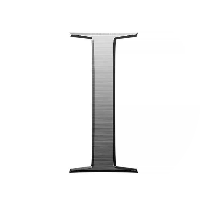# Logic-Based Number Systems (Binary, Octal, Decimal and Hexadecimal)

This quiz contains multiple-choice problems on computer fundamentals subtopics like positional, non-positional, decimal, binary, octal and hexadecimal number systems.

Start Quiz

The base is the total number of digits in a number system. True or false?

True

False

The LSB and MSB of 1243247 are __ and __.

1, 7

4, 7

7, 1

4, 1

__ is a device that uses positional notations to represent a decimal number.

Abacus

Calculator

Pascaline

Computer

The 2’s complement of 5 is

1011

0101

1010

0011

What does BCD stand for?

Bitwise coded decimal

Binary coded decimal

Binary converted decimal

Bitwise converted decimal

One zettabyte =?

1024 TB

1024 EB

1024 ZB

1024 PB

Result of 2 + 3 (in BCD format) =

0010

0011

0101

1010

ASCII stands for

American standard code for international interchange

American scientific code for information interchange

American scientific code for international interchange

American standard code of information interchange

The 2’s complement of 15 is

0000

0001

0010

0100

Another name for the base is

Root

Entity

Median

The decimal equivalent of (0.101)2 will be

0.5

0.625

0.25

0.875

The signed magnitude for -3 will be

00000011

10000011

11111101

11111100

A number with both integer and fractional parts has digits raised to both positive and negative powers of 2 in a decimal number system. True or false?

True

False

Which of the following is not a type of number system?

Positional

Non-Positional

Octal

Fractional

How is the number 5 represented in a non-positional number system?

IIIII

5

V

v

Quiz/Test Summary
Title: Logic-Based Number Systems (Binary, Octal, Decimal and Hexadecimal)
Questions: 15
Contributed by: